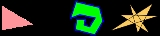xPolygonWordNet

### noun

(1)   A closed plane figure bounded by straight sidesWiktionaryText

### Etymology

< and ; compare French

### Noun

1. A plane figure bounded by straight edges.
2. The boundary of such a figure.
3. A figure comprising vertices and (not necessarily straight) edges, alternatingly.
4. Such a figure and its interior, taken as a whole.

### Noun

polygon c (plural polygoner, definite singular polygonen, definite plural polygonerna)
1. polygon